load("workspace.RData")

## Simulate white Noise

Lets Simulate the ACF of the white noise. it is normally distributed

set.seed(2)
acf(rnorm(100))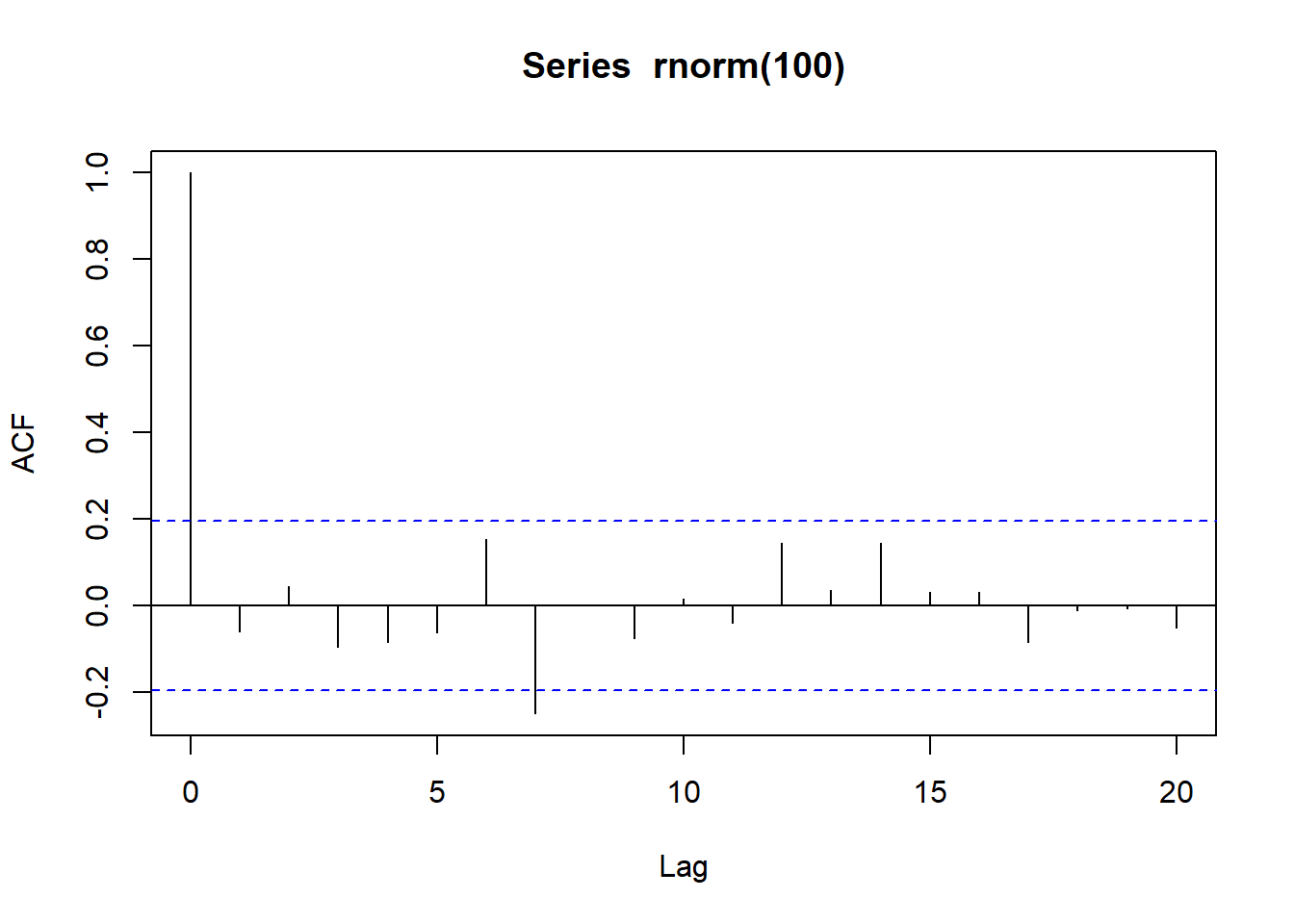Here the statistical significant value at lag =7 is due to sampling variation.

## Simulate Random Walk

x <- w <- rnorm(1000)
for (t in 2:1000)x[t] <- x[t-1]+w[t]
plot(x,type="l")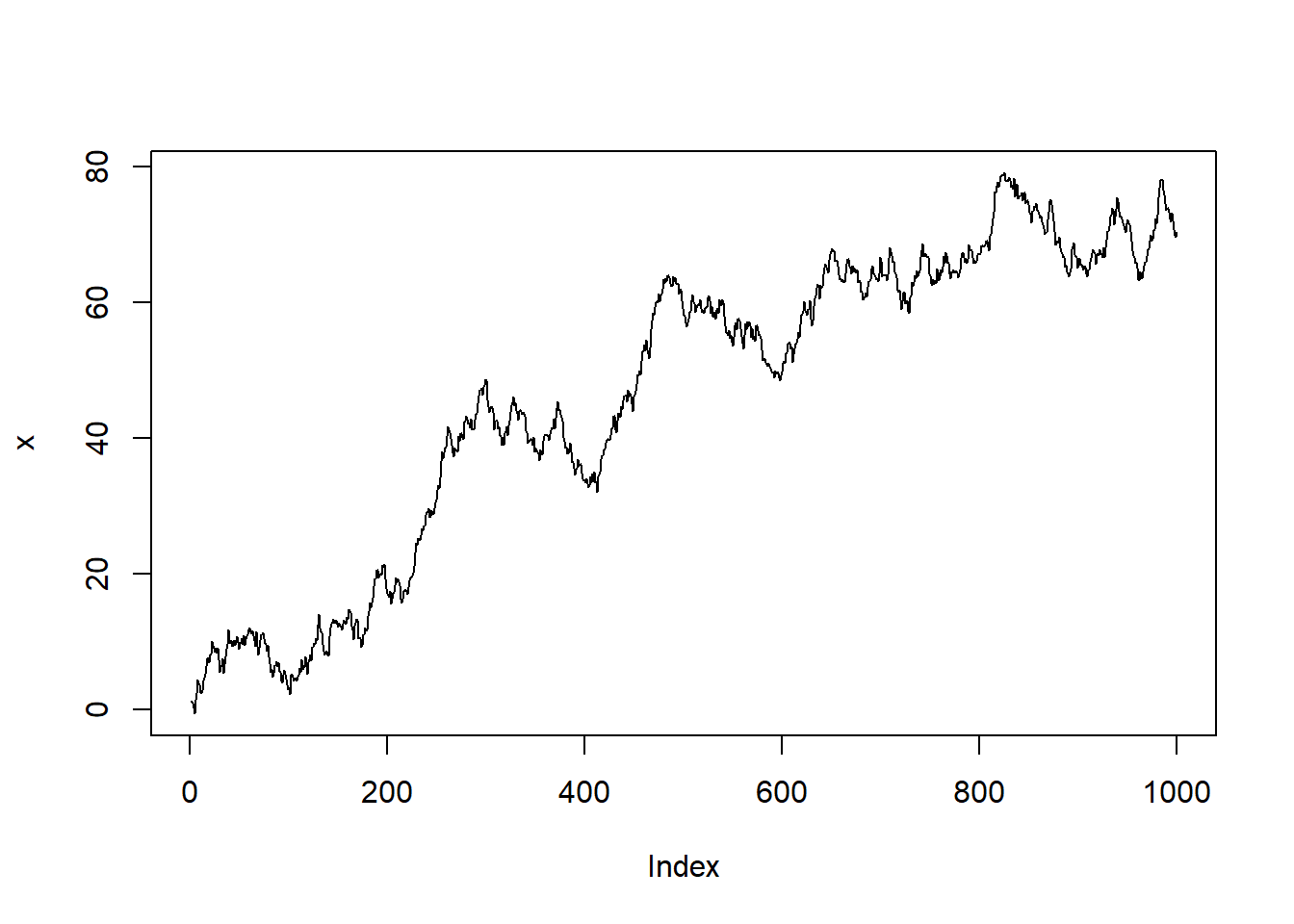The series exhibits an increasing trend. However, this is purely stochastic and due to the high serial correlation.Lets draw its ACF

acf(x)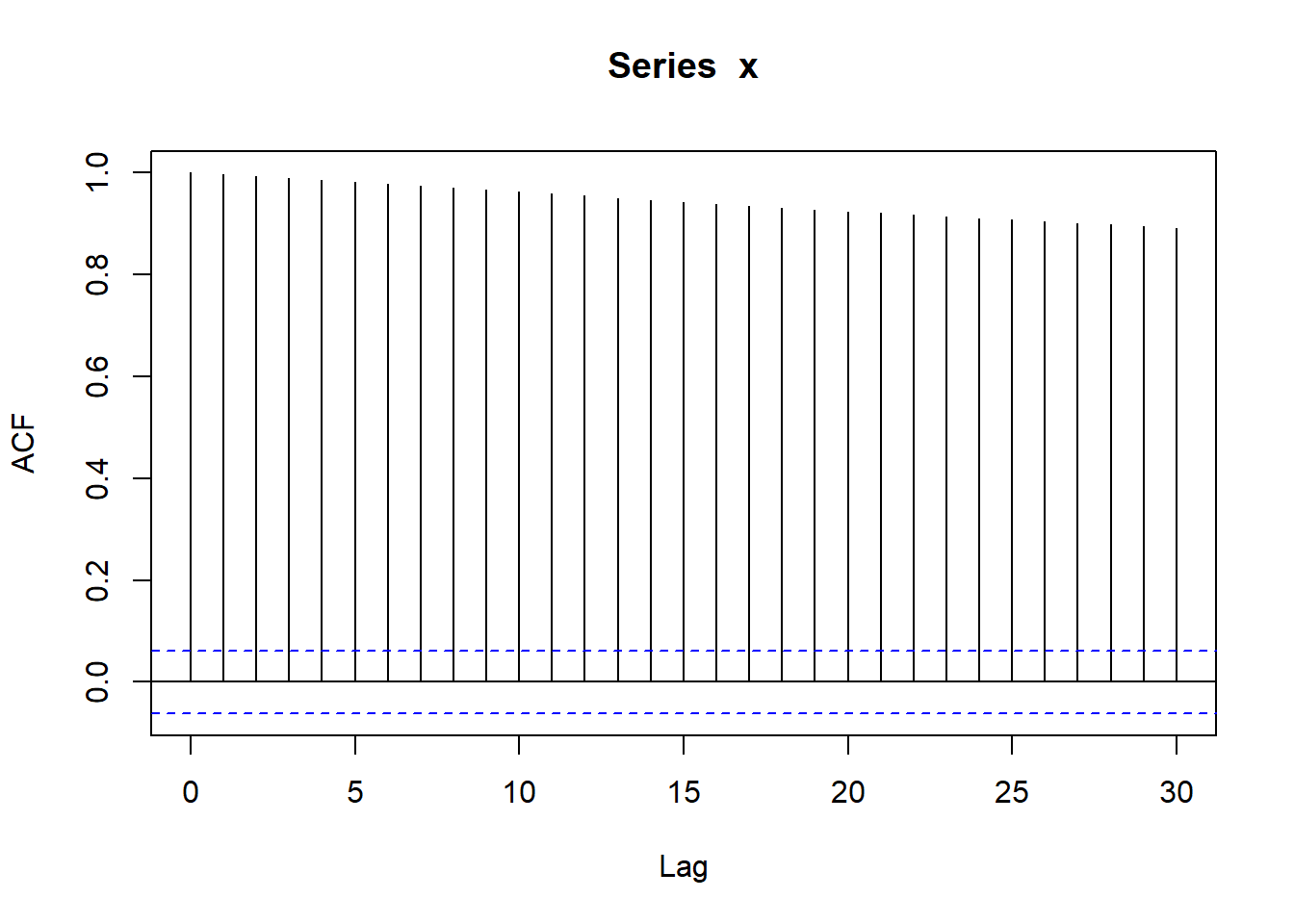As evident, its a gradual decay- its a notable feature of random walk series.

## Fitted Model and Diagnostic Plots

The first order differences of a random walk are a white noise series, so that the correlogram of the series of differences can be used to assess whether a given series is reasonably modeled as a random walk.

acf(diff(x))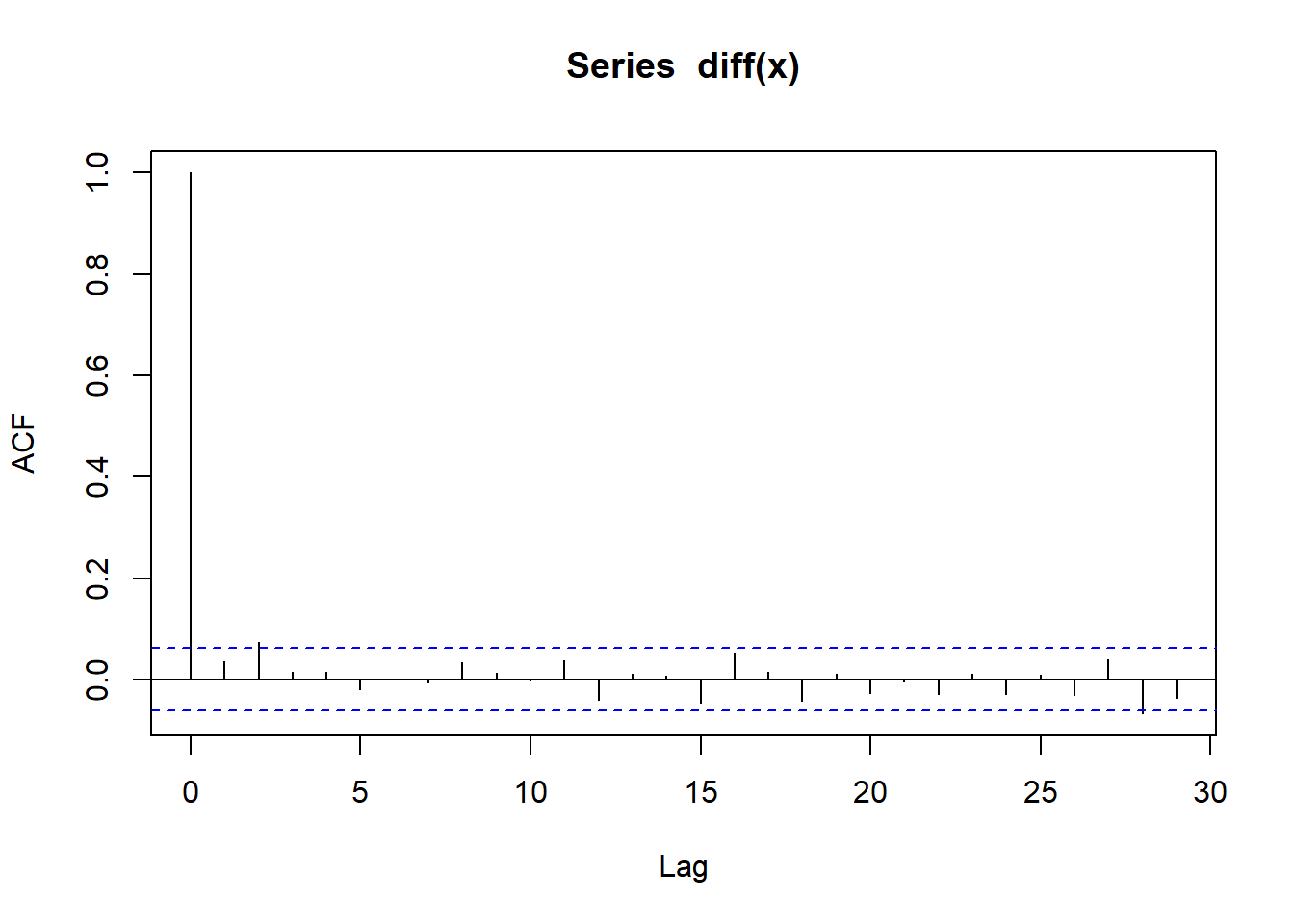So there are no obvious patterns in the correlogram. So If a series follows a random walk, the differenced series will be a white noise.

DP1 <- diff(x)
mean(DP1)+c(-2,2)*sd(DP1)/sqrt(length(DP1))
##  0.006397314 0.132060610

We can check if it is a random walk or random walk with drift by calculating mean of difference of x and the confidence interval. if it contains 0 which it is then, it is random walk. Else it is random walk with drift with the drift of mean(diff(x))

For exchange rate data - lets see the acf of that

acf(diff(Z.ts))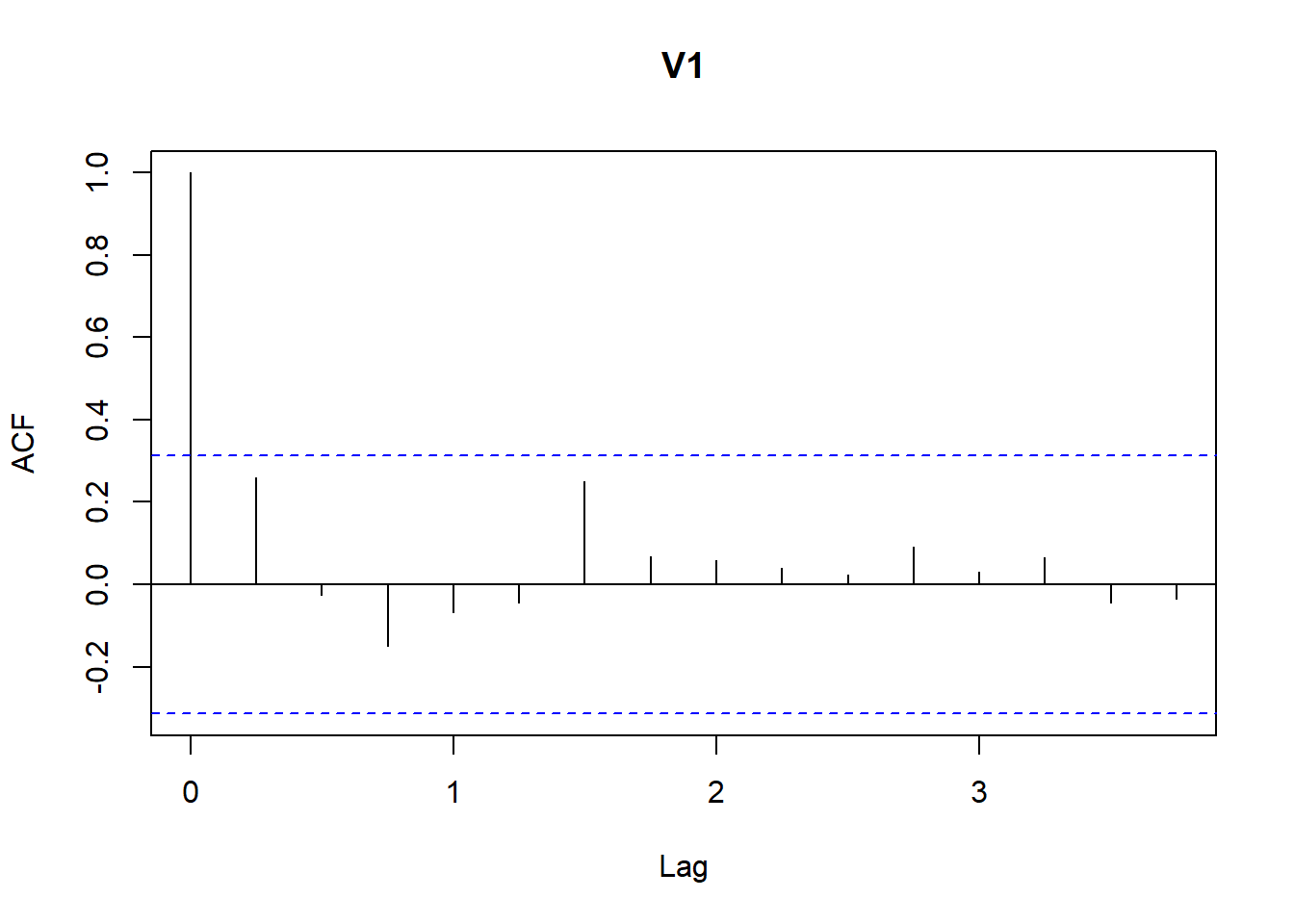Here lag 1 is significant. But the lack of any significant value suggests that the random walk provides a good approximation for the series. Lets use the HW model, we know that it is closer to white noise. So adding beta term while making alpha 1 and gamma as zero or false

Z.hw <- HoltWinters(Z.ts,alpha=1,gamma=FALSE)
acf(resid(Z.hw))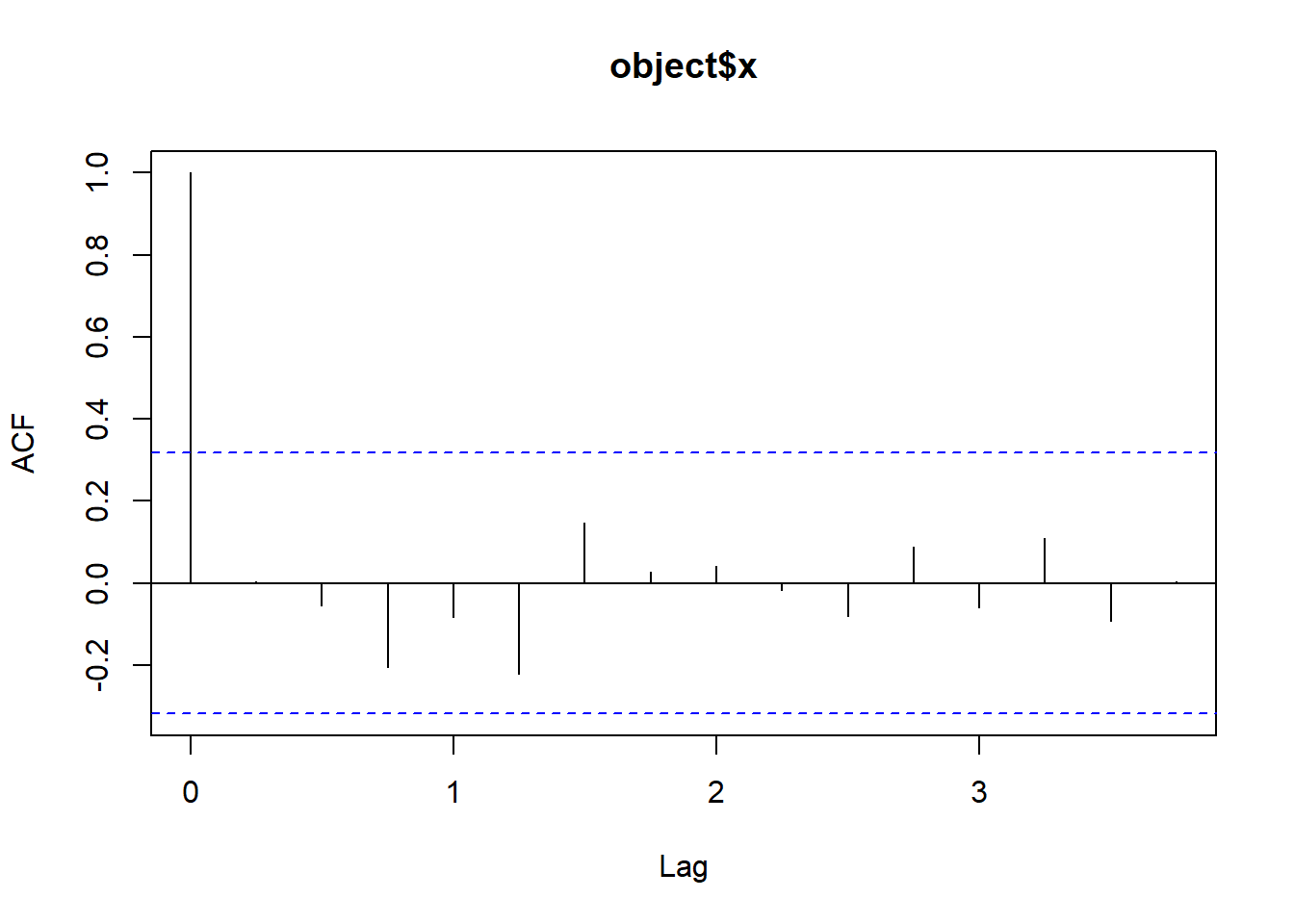So we see that it is much more closer to the white noise.

## Share Price of Hewlett-Packard Stock

• Period: Upto June 7, 2007- 672 trading days.
• Data: values
• Any Transformation in the Data: value
• Type: Closing Price of stock
• Unit: US dollars
• Frequency: Daily
• Start of Frequency:
• End of Frequency: Upto June 7, 2007

Lets read the data and plot

HP.dat <- read.table("ts/HP.txt",header=T)
plot(as.ts(HP.dat$Price))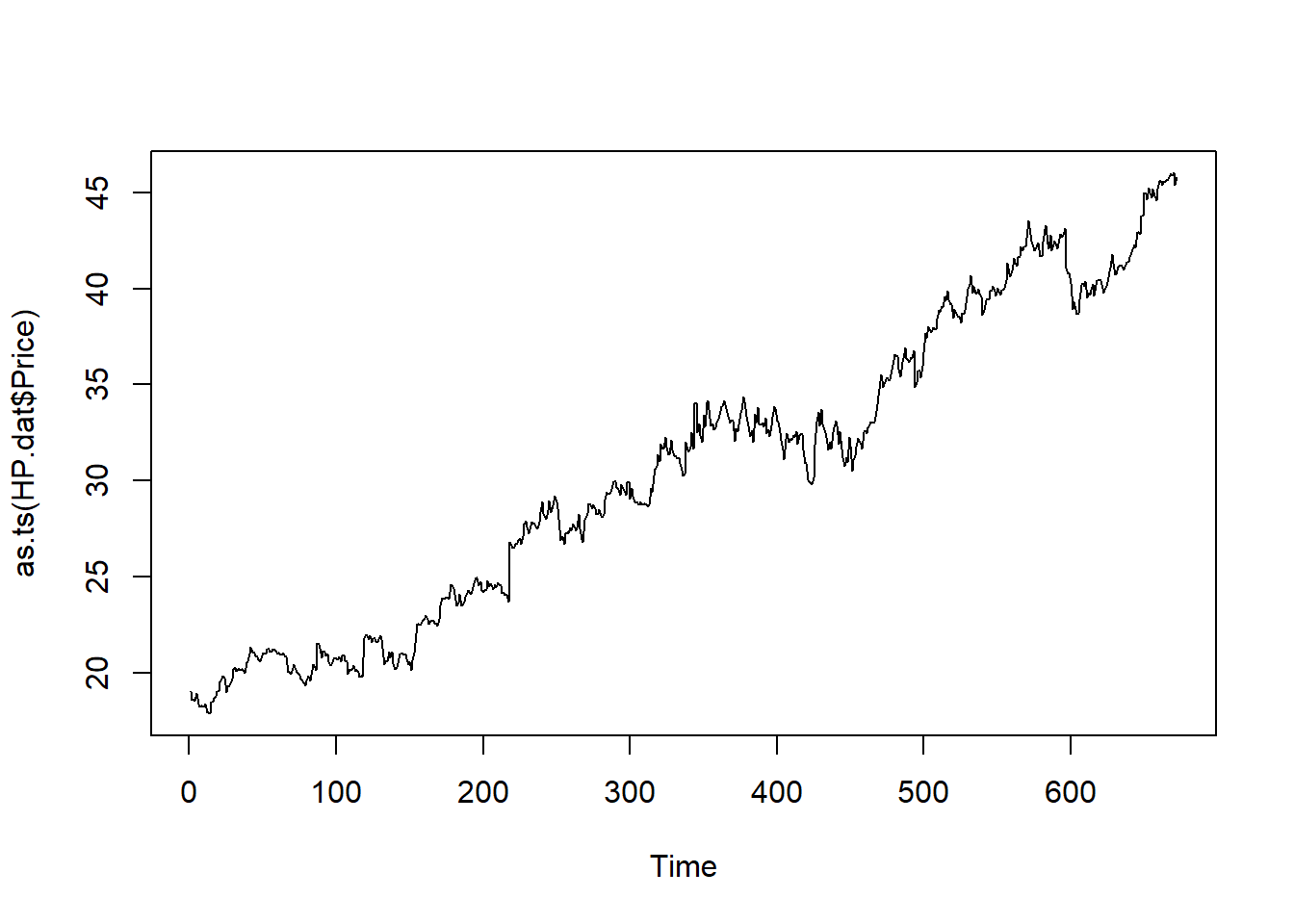lets find the difference and see the nature of the walk DP <- diff(HP.dat$Price)
plot(as.ts(DP))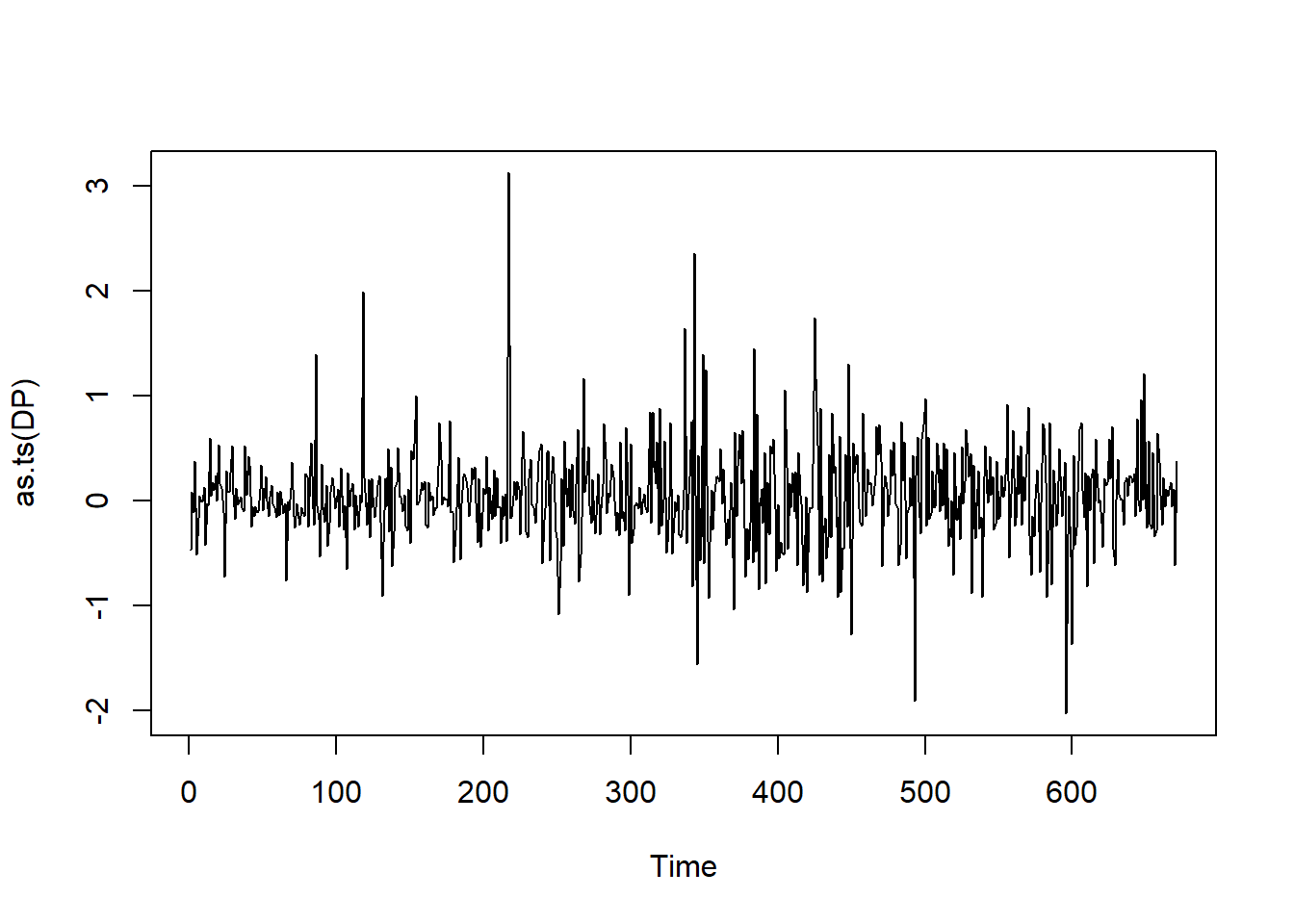lets see the ACF

acf(DP)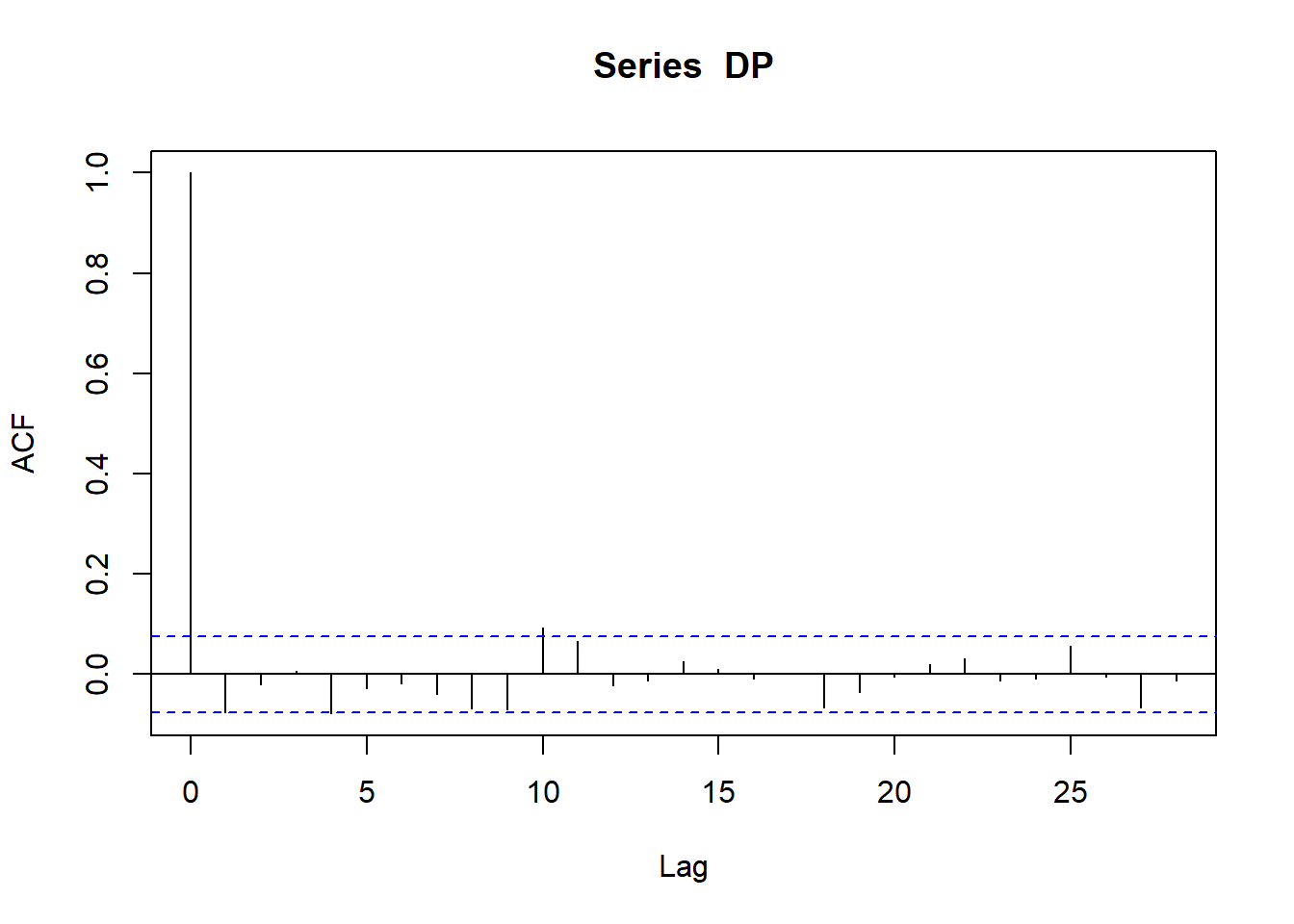correlogram can be well modeled as white noise. Let us see if it is random walk or random walk with drift

mean(DP);
##  0.03986587
mean(DP)+c(-2,2)*sd(DP)/sqrt(length(DP))
##  0.004378275 0.075353468

As the confidence interval doesn’t contain zero, it is random walk with drift with drift value equal to 0.0398.

## Autoregressive Model

The series {Xt} is an autoregressive process of order 1, if Xt= alpha1 * X(t-1) of order 2 if Xt = alpha1 * X(t-1)+ alpha 2 * X(t-2)

and so on. where alpha1, alpha2 are model parameters

## Simulation of AR(1) process

set.seed(1)
x <- w <- rnorm(100)
for (t in 2:100) x[t] <- 0.7 * x[t-1]+w[t]
plot(x,type="l")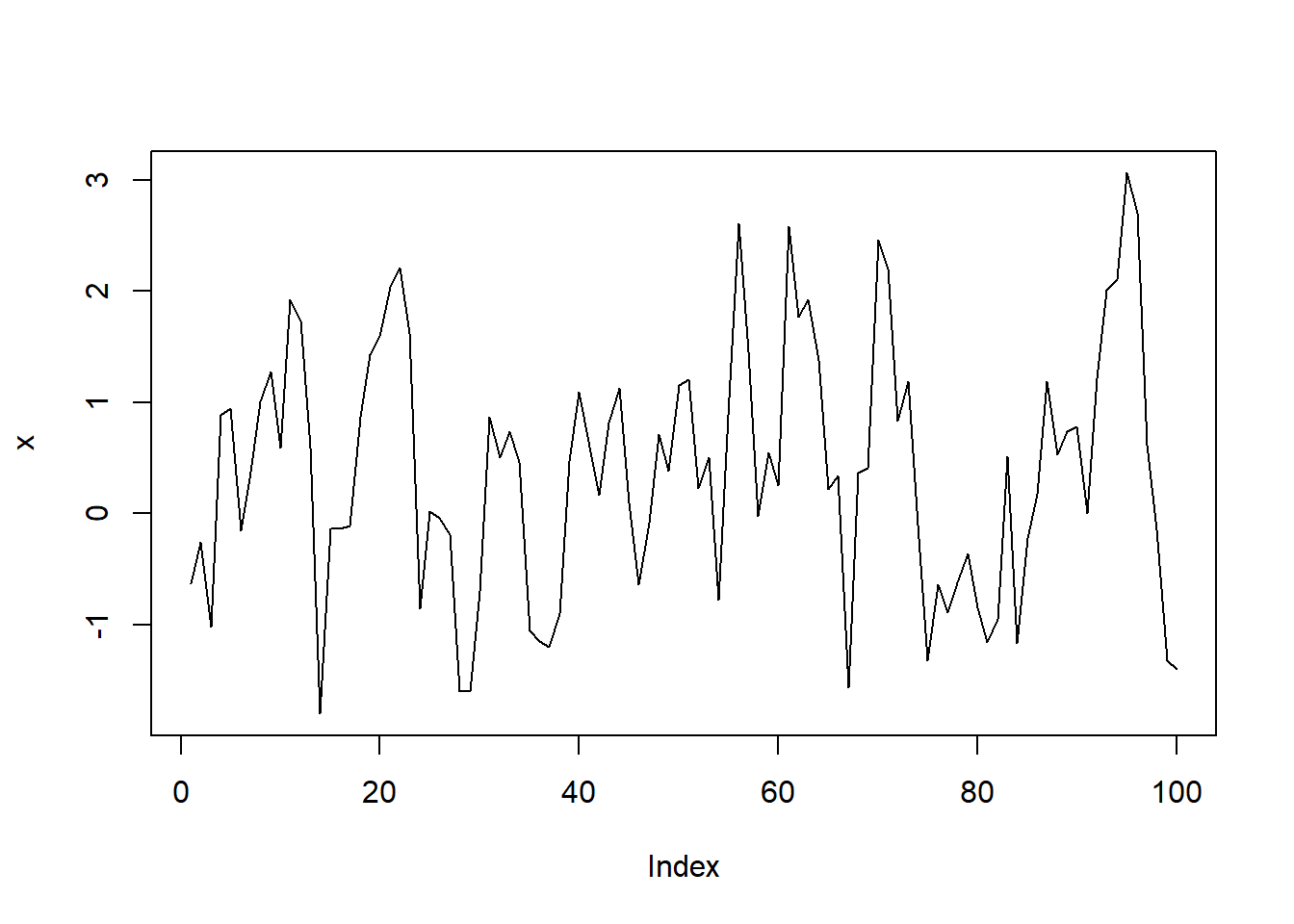Lets see the ACF

acf(x)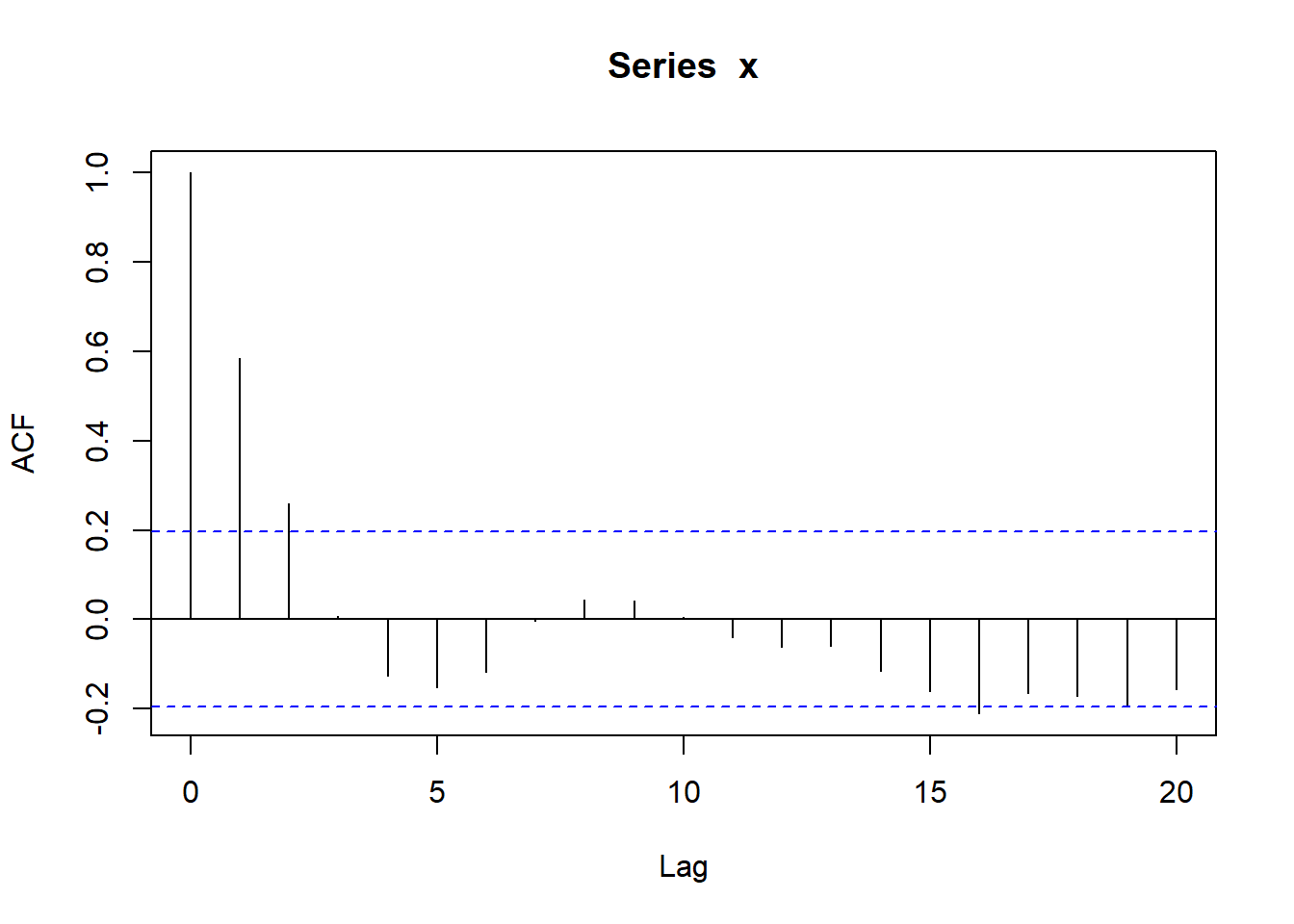PACF. partial correlation at lag k is the correlation that results after removing the effect of any correlation due to terms at shorter lags.

pacf(x)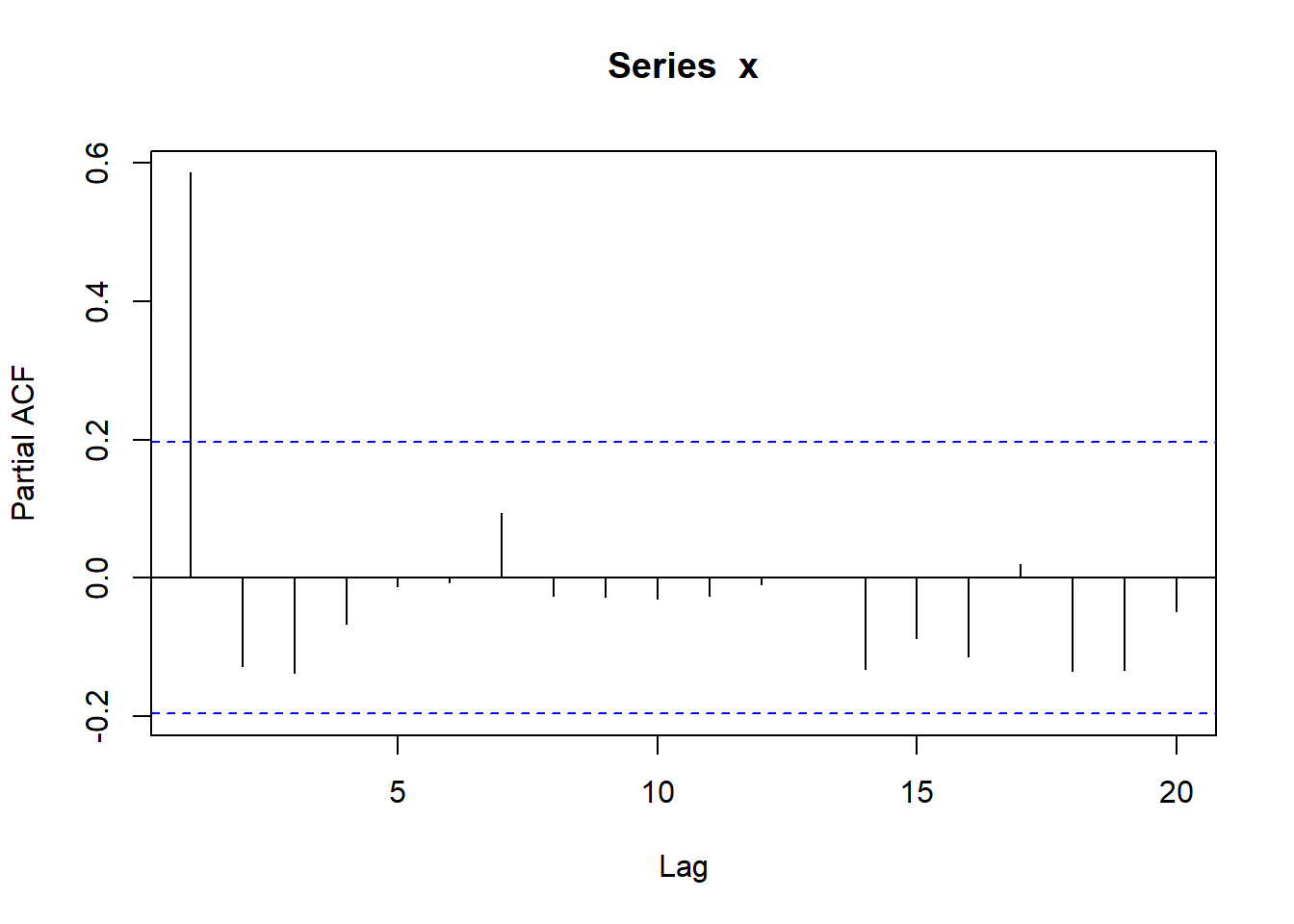The partial correlation has no significant correlation except the value at lag 1. PACF starts at lag 1 while ACF starts at lag 0.

## ar function : To fit an AR model

Lets fit the AR model on our previously used model and estimate the value of alpha. We have set the value of alpha at 0.7, let us see how much model will estimate

set.seed(1)
x <- w <- rnorm(100)
for (t in 2:100) x[t] <- 0.7 * x[t-1]+w[t]

The method MLE is used for fitting purpose

x.ar <- ar(x,method="mle")
x.ar$order ##  1 To it gave us the correct order, which is one. Now let us derive the alpha. x.ar$ar
##  0.6009459

So the value of alpha as per the model is 0.60 let us see if the confidence interval contains 0.70

x.ar$ar+c(2,-2)*sqrt(x.ar$asy.var)
## Warning in c(2, -2) * sqrt(x.ar$asy.var): Recycling array of length 1 in vector-array arithmetic is deprecated. ## Use c() or as.vector() instead. ##  0.7614886 0.4404031 So the value 0.70 is contained in the confidence interval. ## Global Temperature Series: Fitted AR Model We have done this earlier, first lets take out the seasonality with the function aggregate Global.ar <- ar(aggregate(Global.ts,FUN=mean),method="mle") ## Warning in arima0(x, order = c(i, 0L, 0L), include.mean = demean): possible ## convergence problem: optim gave code = 1 Lets find the order Global.ar$ar
##  0.58762026 0.01260254 0.11116731 0.26763656

So it is of the order 4. To test it if indeed it is, we can check the ACF

acf(Global.ar$res[-(1:Global.ar$order)],lag=50)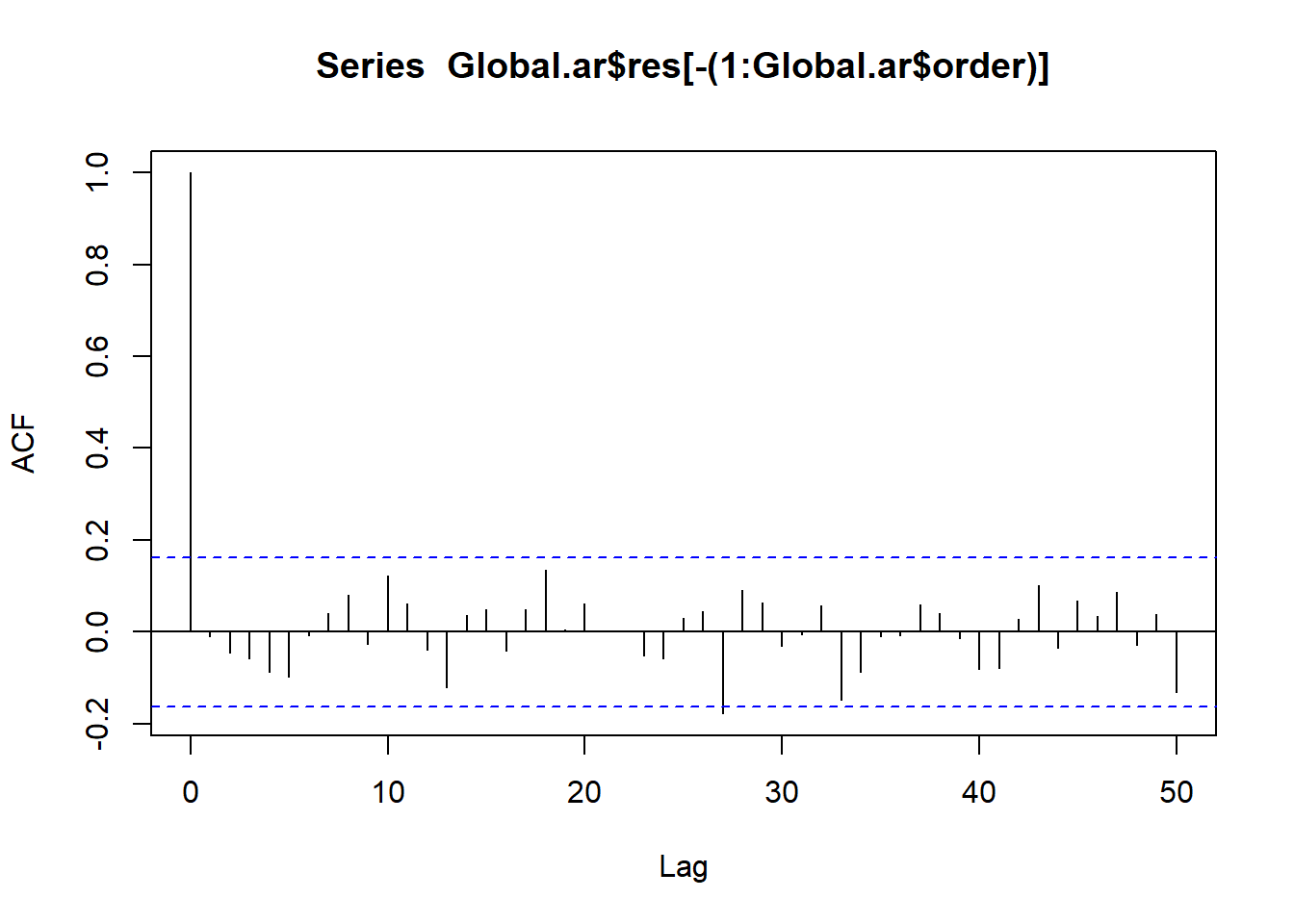The correlogram is approximately white noise so that, in the absence of further information, a simple stochastic model can ‘explain’the correlation and trends in the series.

As the AR model has no deterministic trend component, the trends in the data can be explained by serial correlation and random variation, implying that it is possible that these trends are stochastic (or could arise from a purely stochastic process.

save.image("workspace.Rdata")